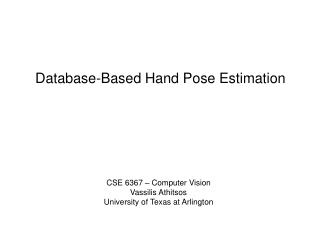# Database-Based Hand Pose Estimation - PowerPoint PPT PresentationDownload PresentationDatabase-Based Hand Pose Estimation

Database-Based Hand Pose EstimationDownload Presentation## Database-Based Hand Pose Estimation

- - - - - - - - - - - - - - - - - - - - - - - - - - - E N D - - - - - - - - - - - - - - - - - - - - - - - - - - -
##### Presentation Transcript

1. Database-Based Hand Pose Estimation CSE 6367 – Computer Vision Vassilis Athitsos University of Texas at Arlington

2. Static Gestures (Hand Poses) Given a hand model, and a single image of a hand, estimate: 3D hand shape (joint angles). 3D hand orientation. Joints Input image Articulated hand model

3. Static Gestures Given a hand model, and a single image of a hand, estimate: 3D hand shape (joint angles). 3D hand orientation. Input image Articulated hand model

4. Given the 3D hand pose in the previous frame, estimate it in the current frame. Problem: no good way to automatically initialize a tracker. Rehg et al. (1995), Heap et al. (1996), Shimada et al. (2001), Wu et al. (2001), Stenger et al. (2001), Lu et al. (2003), … Goal: Hand Tracking Initialization

5. Assumptions in Our Approach A few tens of distinct hand shapes. All 3D orientations should be allowed. Motivation: American Sign Language.

6. Assumptions in Our Approach A few tens of distinct hand shapes. All 3D orientations should be allowed. Motivation: American Sign Language. Input: single image, bounding box of hand.

7. Assumptions in Our Approach We do not assume precise segmentation! No clean contour extracted. input image skin detection segmented hand

8. Approach: Database Search Over 100,000 computer-generated images. Known hand pose. input

9. Why? We avoid direct estimation of 3D info. With a database, we only match 2D to 2D. We can find all plausible estimates. Hand pose is often ambiguous. input

10. Building the Database 26 hand shapes

11. Building the Database 4128 images are generated for each hand shape. Total: 107,328 images.

12. Features: Edge Pixels We use edge images. Easy to extract. Stable under illumination changes. input edge image

13. Chamfer Distance input model Overlaying input and model How far apart are they?

14. Input: two sets of points. red, green. c(red, green): Average distance from each red point to nearest green point. Directed Chamfer Distance

15. Input: two sets of points. red, green. c(red, green): Average distance from each red point to nearest green point. c(green, red): Average distance from each red point to nearest green point. Directed Chamfer Distance

16. Input: two sets of points. red, green. c(red, green): Average distance from each red point to nearest green point. c(green, red): Average distance from each red point to nearest green point. Chamfer Distance Chamfer distance: C(red, green) = c(red, green) + c(green, red)

17. Evaluating Retrieval Accuracy A database image is a correct match for the input if: the hand shapes are the same, 3D hand orientations differ by at most 30 degrees. correct matches input incorrect matches

18. Evaluating Retrieval Accuracy An input image has 25-35 correct matches among the 107,328 database images. Ground truth for input images is estimated by humans. correct matches input incorrect matches

19. Evaluating Retrieval Accuracy Retrieval accuracy measure: what is the rank of the highest ranking correct match? correct matches input incorrect matches

20. Evaluating Retrieval Accuracy input … … rank 1 rank 2 rank 3 rank 4 rank 5 rank 6 highest ranking correct match

21. Results on 703 Real Hand Images

22. Results on 703 Real Hand Images • Results are better on “nicer” images: • Dark background. • Frontal view. • For half the images, top match was correct. 22

23. Examples segmented hand edge image initial image correct match rank: 1

24. Examples segmented hand edge image initial image correct match rank: 644

25. Examples segmented hand edge image initial image incorrect match rank: 1

26. Examples segmented hand edge image initial image correct match rank: 1

27. Examples segmented hand edge image initial image correct match rank: 33

28. Examples segmented hand edge image initial image incorrect match rank: 1

29. Examples segmented hand edge image “hard” case segmented hand edge image “easy” case

30. Research Directions More accurate similarity measures. Better tolerance to segmentation errors. Clutter. Incorrect scale and translation. Verifying top matches. Registration.

31. Efficiency of the Chamfer Distance Computing chamfer distances is slow. For images with d edge pixels, O(d log d) time. Comparing input to entire database takes over 4 minutes. Must measure 107,328 distances. input model

32. The Nearest Neighbor Problem database

33. The Nearest Neighbor Problem Goal: find the k nearest neighbors of query q. database query

34. The Nearest Neighbor Problem Goal: find the k nearest neighbors of query q. Brute force time is linear to: n (size of database). time it takes to measure a single distance. database query

35. Goal: find the k nearest neighbors of query q. Brute force time is linear to: n (size of database). time it takes to measure a single distance. The Nearest Neighbor Problem database query